# Independent Samples Z-Test - Comparing Two Means with Equal Variances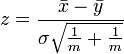$z = \frac{\bar{x} - \bar{y}}{\sigma \sqrt{\frac{1}{m} + \frac{1}{m}}}$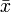$\bar{x}$ and$\bar{x}$ are the average values of variables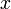$x$ and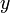$y$ respectively, where each of these variables represents the data from two independent groups,
the groups have sample sizes of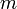$m$ and$n$ respectively,$\sigma = \sqrt{\frac{(m-1)\sigma^2_x + (m-1)\sigma^2_y }{m + n - 2b}}$,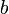$b$ is 1 if Bessel's correction for Means is selected in Statistical Assumptions and 0 otherwise,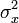$\sigma^2_x$ and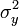$\sigma^2_y$ are the variances in the two groups, and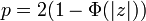$p = 2(1-\Phi(|z|))$